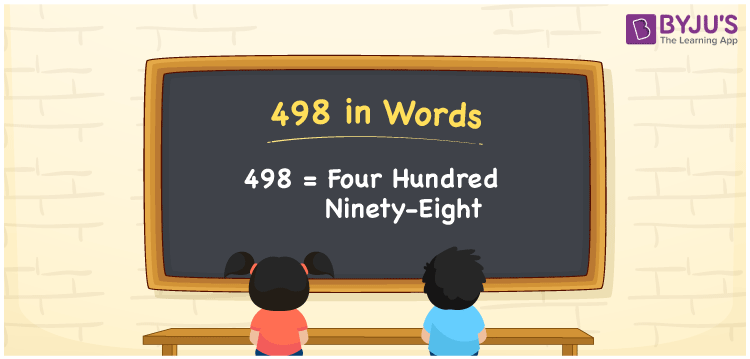# 498 in Words

498 in words is written as Four hundred ninety-eight. In both the International System of Numerals and the Indian System of Numerals, 498 is written as Four hundred ninety-eight. The number 498 is a Cardinal Number as it represents some quantity. For example, “the area of this land is 498 square feet”.

 498 in Words Four hundred ninety-eight Four hundred ninety-eight in Number 498

## 498 in English Words

We write 498 in Words using the letters of the English alphabet. Therefore, we read 498 in English as “Four hundred ninety-eight.”## How to Write 498 in Words?

To write 498 in words, we shall use the place value chart. In the place value chart, write 4 in the hundreds, 9 in the tens, and 8 in the ones, respectively. Now let us make a place value chart to write the number 498 in words.

 Hundreds Tens Ones 4 9 8

Thus, we can write the expanded form as

4 × Hundred + 9 × Ten + 8 × One

= 4 × 100 + 9 × 10 + 8 × 1

= 400 + 90 + 8

= 498

= Four hundred ninety-eight.

498 is a natural number, the successor of 497 and the predecessor of 499.

498 in words – Four hundred ninety-eight

• Is 498 an odd number? – No
• Is 498 an even number? – Yes
• Is 498 a perfect square number? – No
• Is 498 a perfect cube number? – No
• Is 498 a prime number? – No
• Is 498 a composite number? – Yes

## Frequently Asked Questions on 498 in Words

Q1

### How to write 498 in words?

498 in words is written as Four hundred ninety-eight.
Q2

### How to write 498 in the International and Indian System of Numerals?

In both, the system of numerals, 498 in words, is written as Four hundred ninety-eight.
Q3

### What is the preceding number of 498?

The number that precedes 498 is 497.